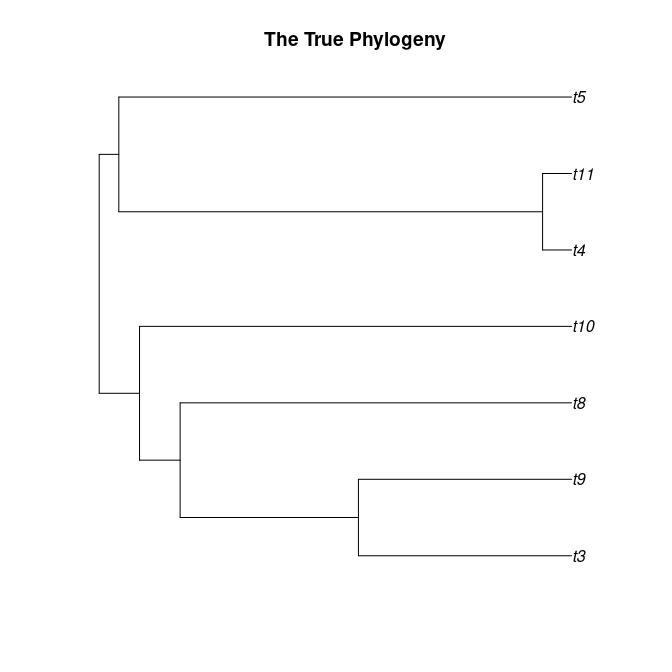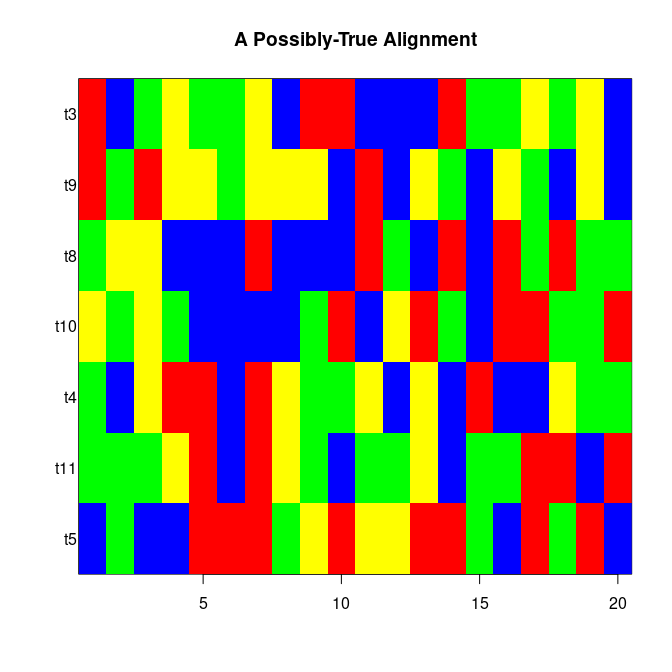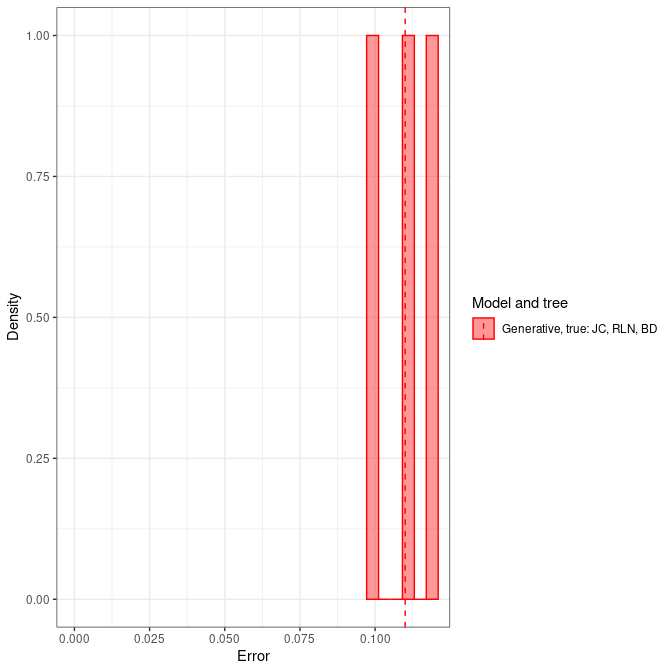# Tutorial

#### 2023-01-19

This vignette demonstrates the pirouette package, with a focus on the research questions that pirouette can answer.

# Main question

The main question that pirouette answers is:

What is the error BEAST2 makes in inferring a given phylogeny?

The same question, but from the point of view of a theoretician:

Would we know the true phylogeny in nature, how well would we be able to infer it from a DNA alignment?

library(pirouette)

# Inferring a simplest world

Let’s assume we know speciation (we don’t) and we assume that speciation is as simple as possible (it is not).

In that simplest world:

• Speciation follows a (constant-rate) birth-death process
• DNA sequences of all species have the same mutation rate
• In DNA mutations, mutation rates between all nucleotides are equal

We can now try to answer pirouette’s main question:

Would we know the true phylogeny in nature, how well would we be able to infer it from a DNA alignment?

Here we go, the true phylogeny of the world:

set.seed(42)
phylogeny <- create_exemplary_dd_tree(n_taxa = 6)
ape::plot.phylo(main = "The True Phylogeny", phylogeny)This phylogeny cannot be measured in nature directly. Instead, we can measure (and align) the DNA sequences of our species.

pirouette needs an approximate idea of how an alignment in nature behaves.

What we do know (or: assumed in our simples world) is (1) that the DNA sequences of all species have the same mutation rate, and (2) In DNA mutations, mutation rates between all nucleotides are equal. This amounts to (1) the clock model is a strict clock model, and (2) the site model is a Jukes-Cantor model.

What we need to have approximately right is a mutation rate, which must not be too low, nor too high. A mutation rate that is too low (e.g. zero) would result in an uninformative alignment (e.g. all species would have the same sequence). A mutation rate that is too high, results in uninformative alignments as well, consisting of random noise.

What we do for convenience is specify an ancestral DNA sequence that is simple and easy to interpret visually, as well as an RNG seed:

alignment_params <- pirouette::create_alignment_params(
root_sequence = create_blocked_dna(length = 20),
rng_seed = 314
)
print(alignment_paramsroot_sequence) #>  "aaaaacccccgggggttttt" From here, we can already predict the DNA alignment pirouette will generate: alignment <- create_true_alignment( true_phylogeny = phylogeny, alignment_params = alignment_params ) ape::image.DNAbin( alignment, main = "A Possibly-True Alignment", legend = FALSE )Back to our main question: Would we know the true phylogeny in nature, how well would we be able to infer it from a DNA alignment? To infer a phylogeny from an alignment, we need: • a way to select inference parameters • a general setup for our inference We select our inference parameters based on our knowledge of the generative model. The generative model is the model we followed in simulating the DNA alignment. We do need to specify a tree prior, we we know, due to our knowledge of The Simplest World. type run_if measure evidence inference model generative always FALSE Default experiment <- create_experiment( inference_conditions = create_inference_conditions( model_type = "generative", run_if = "always", do_measure_evidence = FALSE ), inference_model = create_inference_model( tree_prior = create_bd_tree_prior(), mcmc = create_test_mcmc() ), beast2_options = create_beast2_options(rng_seed = 314) ) experiments <- list(experiment) The general setup for our inference can be tuned. For example, to have a shorter MCMC run and a specific RNG seed: Now we have all in place to do a pirouette run: df <- NULL if (rappdirs::app_dir()os != "win" && beastier::is_beast2_installed()) {

df <- pirouette::pir_run(
phylogeny = phylogeny,
pir_params = create_pir_params(
alignment_params = alignment_params,
experiments = experiments
)
)
} else {
df <- create_test_pir_run_output()
}
knitr::kable(df)
tree inference_model inference_model_weight site_model clock_model tree_prior error_1 error_2 error_3
true generative 0.5 JC69 relaxed_log_normal birth_death 0.1 0.11 0.12

Or in a plot:

pirouette::pir_plot(df)# Doing inference with the best tree prior

From the Simplest World scenarion, we drop one piece of knowledge: we assumre we do not know the tree prior anymore. We do still know The True Phylogeny, but do not know the speciation model that generated it (even though we generated the phylogeny using a birth-death model).

Would we know the true phylogeny in nature, how well would we be able to infer it from a DNA alignment?

So, again, here is the true phylogeny:

ape::plot.phylo(main = "The True Phylogeny", phylogeny)Also, the alignment it generated in the Simplest World as well is still equally valid:

ape::image.DNAbin(
alignment,
main = "A Possibly-True Alignment",
legend = FALSE
)Because we do not know the true tree prior, we instruct pirouette to use the best model to do inference. This best model is determined on the evidence (also: marginal likelihood) for a model in generating the alignment. We can specify the set of models pirouette can pick from. In this case, we let pirouette pick from all (only two!) model combinations of one site model (JC69), one clock model (strict) and two tree priors. We choose pirouette to prefer a quick calculation over a correct estimation by setting epsilon to a high value:

type run_if measure evidence inference model
candidate best_candidate TRUE Yule
candidate best_candidate TRUE Birth-Death
if (rappdirs::app_dir()$os != "win" && beastier::is_beast2_installed()) { mcmc <- create_test_mcmc() experiment_yule <- create_test_cand_experiment() experiment_bd <- create_test_cand_experiment( inference_model = create_inference_model( tree_prior = create_bd_tree_prior(), ) ) # Candidat experiments must produce the same files experiment_yule$beast2_options <- experiment_bd$beast2_options experiment_yule$inference_model$mcmc <- experiment_bd$inference_model$mcmc experiment_yule$errors_filename <- experiment_bderrors_filename experiments <- list(experiment_yule, experiment_bd) check_experiments(experiments) pir_params <- create_pir_params( alignment_params = alignment_params, experiments = experiments, evidence_filename = get_temp_evidence_filename() ) } Now we again have all in place to do a pirouette run: if (rappdirs::app_dir()os != "win" &&
is_beast2_installed() &&
is_beast2_ns_pkg_installed()) {
df <- pirouette::pir_run(
phylogeny = phylogeny,
pir_params = pir_params
)
knitr::kable(df)
pirouette::pir_plot(df)
}